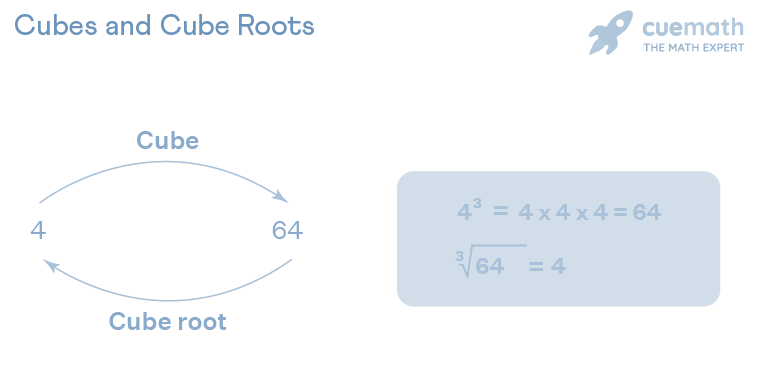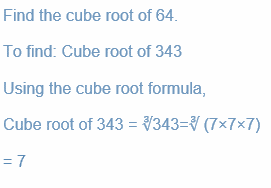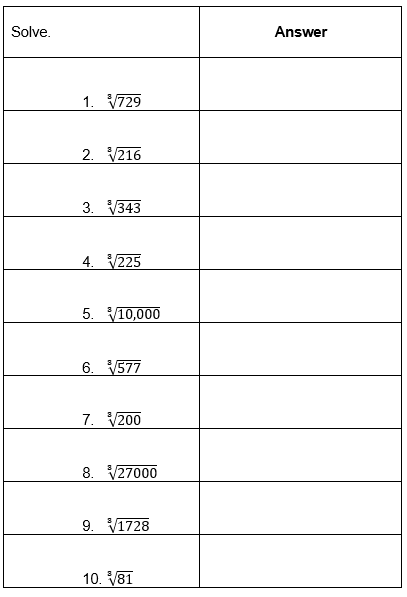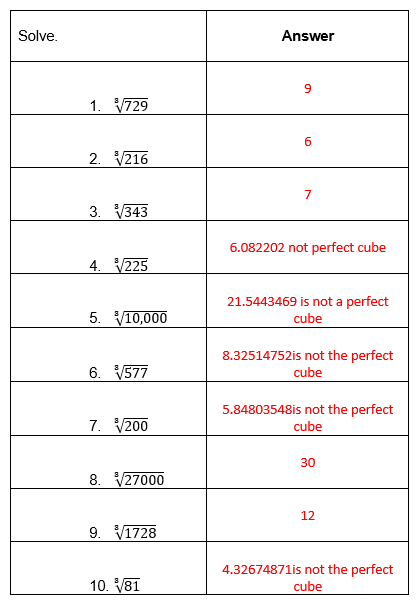# Cube Roots Worksheet (with answer key + PDF)

When we multiply a factor three times to obtain a number, we have obtained the cube root of that factor. 3 cube roots of, the end cube root are used as the cube root symbol. In contrast to cubing a number, finding its cube root is a mathematical operation.

## What is the “Cube Roots Worksheet (with answer key + PDF)”?

This worksheet will explore some of the Cube Roots.

What are Cube Roots?

In mathematics, the definition of cube root is written as Cube root is the number that needs to be multiplied three times to get the original number.

This worksheet will help the students to understand the concept of the particular topic and also this worksheet provides an informative lesson.

Instructions on how to use the “Cube Roots Worksheet (with answer key + PDF).”

Study the concept and examples given and try to solve the given exercises below.

## Conclusion

In mathematics, a number “a” has a number “b” as its cube root, with the result that b3 = a. It implies that a number’s cube root produces a value that, when squared, yields the original number.

If you have any inquiries or feedback, please let us know.

## Cube Roots Worksheet (with answer key + PDF)

Definition of Cube Roots

In mathematics, the definition of cube root is written as Cube root is the number that needs to be multiplied three times to get the original number. Now, let us look at the cube root formula, where y is the cube root of x. ∛x = y. The radical sign ∛ is used as a cube root symbol for any number with a small 3 written on the top left of the sign. Another way to denote a cube root is to write 1/3 as the exponent of a number.How to Find the Cube Root of a Number?

The cube root of a number can be determined by using the prime factorization method. In order to find the cube root of a number:

• Step 1: Start with the prime factorization of the given number.
• Step 2: Then, divide the factors obtained into groups containing three same factors.
• Step 3: After that, remove the cube root symbol and multiply the factors to get the answer. If there is any factor left that cannot be divided equally into groups of three, that means the given number is not a perfect cube and we cannot find the cube root of that number.

The formula to calculate the cube root is given:

• Cube root of x = ∛x = ∛ (y × y × y) = y

Example:## Worksheet

Practice the problems.